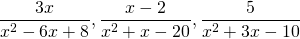Chapter 8: Rational Expressions

# 8.3 Least Common Denominators

Finding the least common denominator, or LCD, is very important to working with rational expressions. The process used depends on finding what is common to each rational expression and identifying what is not common. These common and not common factors are then combined to form the LCD.

Example 8.3.1

Find the LCD of the numbers 12, 8, and 6.

First, break these three numbers into primes: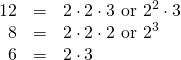Then write out the primes for the first number, 12, and set the LCD to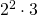.

Notice the factorization of 8 includes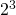, yet the LCD currently only has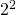, so you add one 2.

Now the LCD =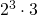.

Checking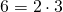, there already is a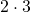in the LCD, so we need not add any more primes.

The LCD =or 24.

This process can be duplicated with variables.

Example 8.3.2

Find the LCD of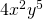and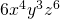.

First, break both terms into primes: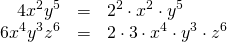Then write out the primes for the first term,, and set the LCD to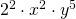.

The LCD for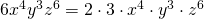has an extra 3,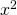, and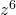, which we add to the LCD that we are constructing.

This yields LCD =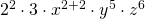, or LCD =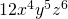.

This process can also be duplicated with polynomials.

Example 8.3.3

Find the LCD of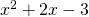and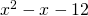.

First, we factor both of these polynomials, much like finding the primes of the above terms: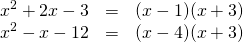The LCD is constructed as we did before, except this time, we write out the factored terms from the first polynomial, so the LCD =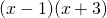.

Notice that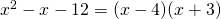, where the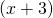is already in the LCD, which means that we only need to add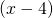.

The LCD =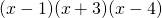.

# Questions

For Questions 1 to 10, find each Least Common Denominator.

1.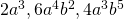2.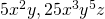3.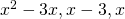4.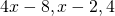5.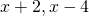6.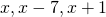7.8.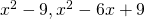9.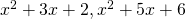10.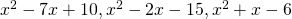For Questions 11 to 20, find the LCD of each fraction and place each expression over the same common denominator.

1.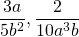2.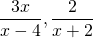3.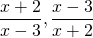4.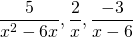5.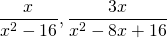6.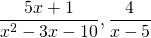7.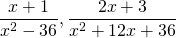8.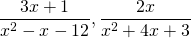9.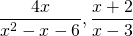10.